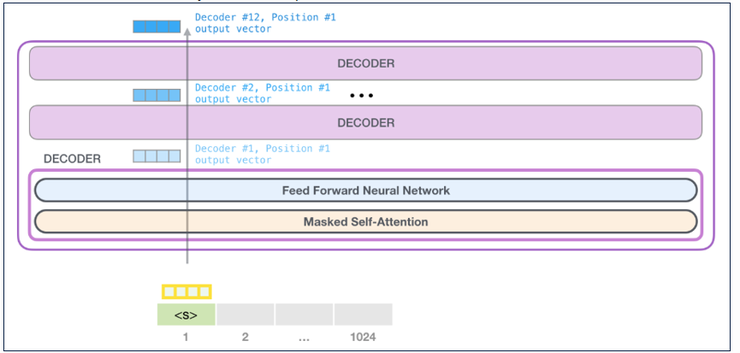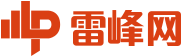# GPT-2没什么神奇的，PyTorch 就可以复现代码

2020/02/25 09:18

——Jeffrey Friedl 在《Mastering Regular Expressions》一书中写道

GPT-2 一开始看起来像是魔术，它的看起来太美丽了，但希望我能为你解释魔术，在你读完这篇文章时揭示所有的技巧，这是我的目标。使那些热衷于理解 GPT-2 模型是如何工作的人更好理解。

——Gordon B. Hinckley

Zero-shot 设置是不微调语言模型并直接在目标数据集上运行推理的设置。例如，在 WebText 上预览一个 LM，并直接尝试预测 Amazon 影评数据集的下一个单词。import torch
import copy
import torch.nn as nn
import torch.nn.functional as F
from torch.nn.modules import ModuleList
from torch.nn.modules.normalization import LayerNorm
import numpy as np
import os
from tqdm import tqdm_notebook, trange
import logging
logging.basicConfig(level = logging.INFO)
logger = logging.getLogger()

GPT-2 内部的 transformer 解码器class Conv1D(nn.Module):
def __init__(self, nx, nf):        super().__init__()
self.nf = nf
w = torch.empty(nx, nf)
nn.init.normal_(w, std=0.02)
self.weight = nn.Parameter(w)
self.bias = nn.Parameter(torch.zeros(nf))

def forward(self, x):
size_out = x.size()[:-1] + (self.nf,)
x = torch.addmm(self.bias, x.view(-1, x.size(-1)), self.weight)
x = x.view(*size_out)
return x

CONV1D 层解释

CONV1D 层本身可以看作是一个线性层。本质上，它是投射一个初始张量 x（最终尺寸为 x.size(-1)）并传递给它，最终尺寸为 self.nf。

d_model = 768
conv1d  = Conv1D(d_model, d_model*3)
x       = torch.rand(1,4,d_model) #represents a sequence of batch_size=1, seq_len=4 and embedding_sz=768, something like "Hello how are you"
x       = conv1d(x)
x.shape

>> torch.Size([1, 4, 2304])

query, key, value = x.split(d_model, dim=-1)

query.shape, key.shape, value.shape
>> (torch.Size([1, 4, 768]), torch.Size([1, 4, 768]), torch.Size([1, 4, 768]))

class FeedForward(nn.Module):
def __init__(self, dropout, d_model=768, nx=768*4):
super().__init__()
self.c_fc    = Conv1D(d_model, nx)
self.c_proj  = Conv1D(nx, d_model)
self.act     = F.gelu
self.dropout = nn.Dropout(dropout)

def forward(self, x):
return self.dropout(self.c_proj(self.act(self.c_fc(x))))class Attention(nn.Module):
super().__init__()
self.d_model = d_model
self.c_attn  = Conv1D(d_model, d_model*3)
self.scale   = scale
self.softmax = nn.Softmax(dim=-1)
self.register_buffer("bias", torch.tril(torch.ones(n_ctx, n_ctx)).view(1, 1, n_ctx, n_ctx))
self.dropout = nn.Dropout(0.1)
self.c_proj  = Conv1D(d_model, d_model)

"return shape [`batch`, `head`, `sequence`, `features`]"
x = x.view(*new_shape)
return x.permute(0, 2, 1, 3)

def _attn(self, q, k, v, attn_mask=None):
scores  = torch.matmul(q, k.transpose(-2, -1))
if self.scale: scores = scores/math.sqrt(v.size(-1))
nd, ns  = scores.size(-2), scores.size(-1)
scores  = self.softmax(scores)
scores  = self.dropout(scores)
outputs = torch.matmul(scores, v)
return outputs

x         = x.permute(0, 2, 1, 3).contiguous()
new_shape = x.size()[:-2] + (x.size(-2)*x.size(-1),)
return x.view(*new_shape)

def forward(self, x):
x        = self.c_attn(x) #new `x` shape - `[1,3,2304]`
q, k, v  = x.split(self.d_model, dim=2)
out      = self._attn(q, k, v)
out      = self.c_proj(out)
return outtransformer 解码器块说明

class TransformerBlock(nn.Module):
super(TransformerBlock, self).__init__()
self.feedforward = FeedForward(dropout=0.1, d_model=768, nx=768*4)
self.ln_1        = LayerNorm(d_model)
self.ln_2        = LayerNorm(d_model)

def forward(self, x):
x = x + self.attn(self.ln_1(x))
x = x + self.feedforward(self.ln_2(x))
return x

transformer 组由注意力层和前馈层组成，如 GPT-2 架构模型规范所述：层规范化被移动到每个子块的输入，这里的子块是注意力和前馈。

GPT-2架构说明

def _get_clones(module, n):
return ModuleList([copy.deepcopy(module) for i in range(n)])

class GPT2(nn.Module):
def __init__(self, nlayers=12, n_ctx=1024, d_model=768, vcb_sz=50257):
super(GPT2, self).__init__()
self.nlayers = nlayers
self.h       = _get_clones(block, 12)
self.wte     = nn.Embedding(vcb_sz, d_model)
self.wpe     = nn.Embedding(n_ctx, d_model)
self.drop    = nn.Dropout(0.1)
self.ln_f    = LayerNorm(d_model)
self.out     = nn.Linear(d_model, vcb_sz, bias=False)
self.loss_fn = nn.CrossEntropyLoss()
self.init_weights()

def init_weights(self):
self.out.weight = self.wte.weight
self.apply(self._init_weights)

def _init_weights(self, module):
if isinstance(module, (nn.Linear, nn.Embedding, Conv1D)):
module.weight.data.normal_(mean=0.0, std=0.02)
if isinstance(module, (nn.Linear, Conv1D)) and module.bias is not None:
module.bias.data.zero_()
elif isinstance(module, nn.LayerNorm):
module.bias.data.zero_()
module.weight.data.fill_(1.0)

def forward(self, src, labels=None, pos_ids=None):
if pos_ids is None: pos_ids = torch.arange(0, src.size(-1)).unsqueeze(0)
inp = self.drop((self.wte(src)+self.wpe(pos_ids)))
for i in range(self.nlayers): inp = self.h[i](inp)
inp     = self.ln_f(inp)
logits  = self.out(inp)
outputs = (logits,) + (inp,)

if labels is not None:
shift_logits = logits[..., :-1, :].contiguous()
shift_labels = labels[..., 1:].contiguous()
loss = self.loss_fn(shift_logits.view(-1, shift_logits.size(-1)), shift_labels.view(-1))
outputs = (loss,) + outputs
return outputs
return logitsmodel = GPT2()
# load pretrained_weights from hugging face

model_dict = model.state_dict() #currently with random initialization

old_keys = []
new_keys = []
for key in state_dict.keys():
if "mlp" in key: #The hugging face state dict references the feedforward network as mlp, need to replace to `feedforward` be able to reuse these weights
new_key = key.replace("mlp", "feedforward")
new_keys.append(new_key)
old_keys.append(key)

for old_key, new_key in zip(old_keys, new_keys):
state_dict[new_key]=state_dict.pop(old_key)

pretrained_dict = {k: v for k, v in state_dict.items() if k in model_dict}

model_dict.update(pretrained_dict)
model.eval() #model in inference mode as it's now initialized with pretrained weights

from transformers import GPT2Tokenizer
tokenizer = GPT2Tokenizer.from_pretrained("gpt2")
context   = torch.tensor([tokenizer.encode("The planet earth")])

def generate(context, ntok=20):
for _ in range(ntok):
out = model(context)
logits = out[:, -1, :]
indices_to_remove = logits < torch.topk(logits, 10)[..., -1, None]
logits[indices_to_remove] = np.NINF
next_tok = torch.multinomial(F.softmax(logits, dim=-1), num_samples=1).squeeze(1)
context = torch.cat([context, next_tok.unsqueeze(-1)], dim=-1)
return context

out = generate(context, ntok=20)
tokenizer.decode(out)

>> 'The planet earth is the source of all of all the light," says the study that the government will'

class Attention_FASTAI(nn.Module):
super().__init__()
self.softmax  = nn.Softmax(dim=-1)
self.scale    = scale
self.atn_drop = nn.Dropout(0.1)
bias=bias) for o in range(3)]

x = layer(x)

def _attn(self, q, k, v, attn_mask=None):
scores  = torch.matmul(q, k.transpose(-2, -1))
if self.scale: scores = scores/math.sqrt(v.size(-1))
attn_prob  = self.atn_drop(self.softmax(scores))
attn_vec   = attn_prob @ v
return attn_vec

x         = x.permute(0, 2, 1, 3).contiguous()
return x.view(bs, seq_len, -1)

def forward(self, q, k, v, mask=None):
bs, seq_len = q.size(0), q.size(1)
wq, wk, wv  = map(lambda o:self.split_heads(*o, bs),
zip((q,k,v), (self.wq, self.wk, self.wv)))
attn_vec    = self._attn(wq, wk, wv)
return attn_vec### GPT-2没什么神奇的，PyTorch 就可以复现代码